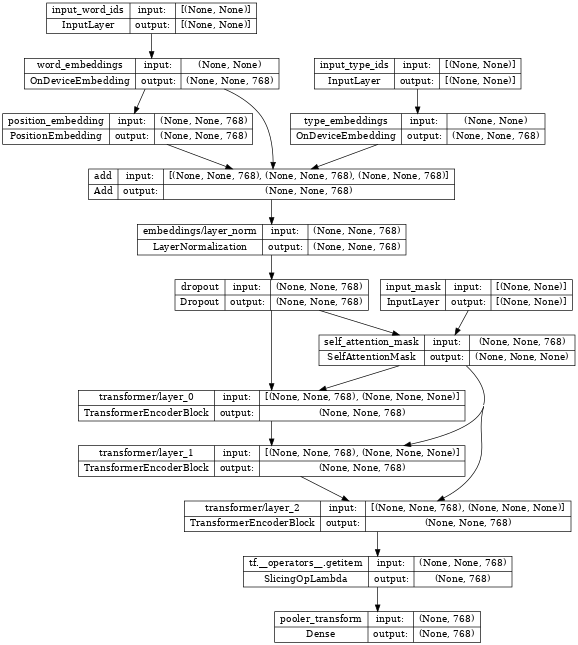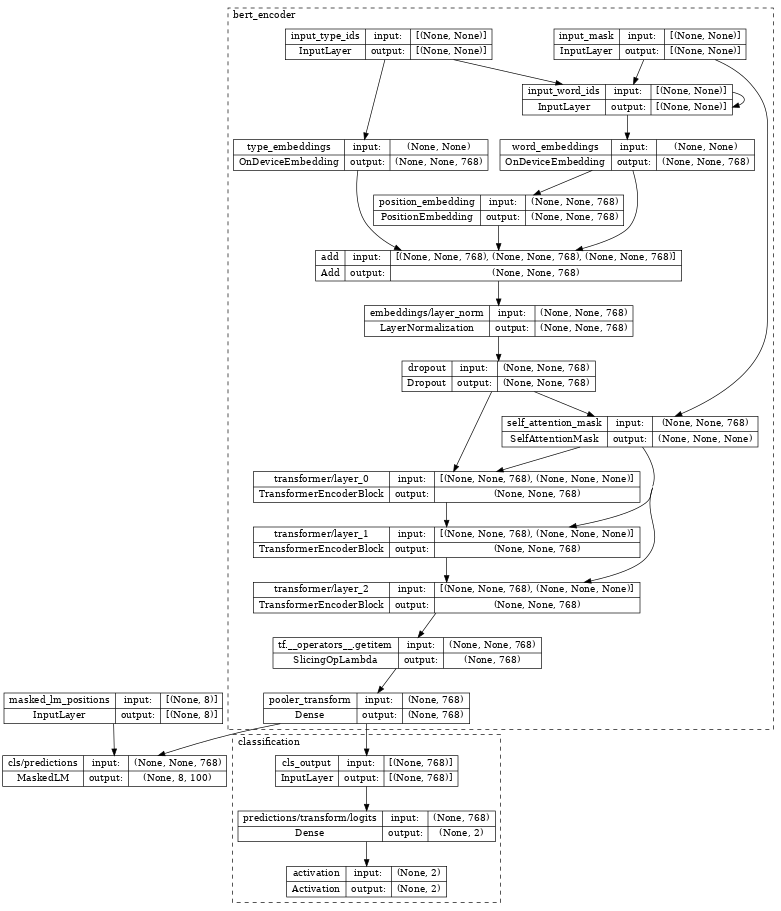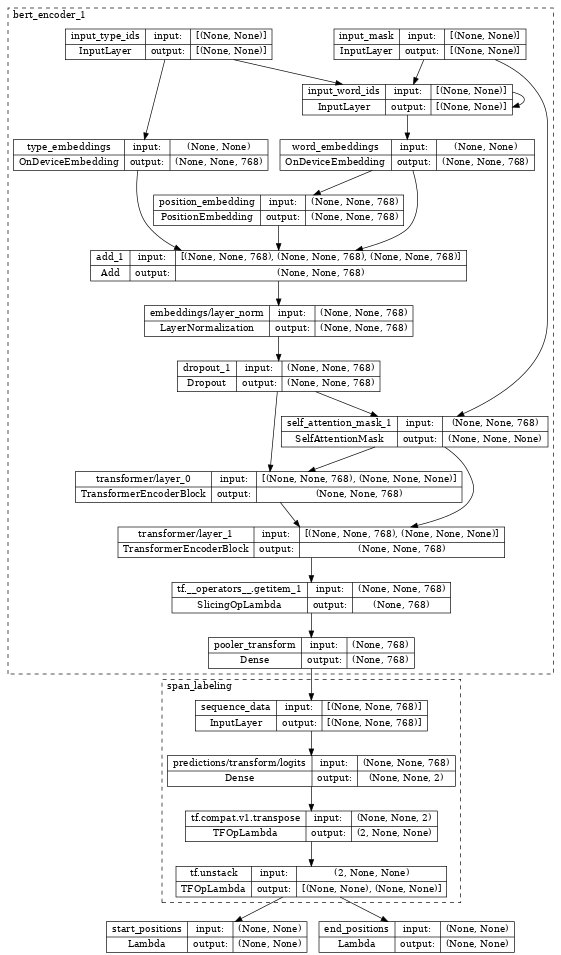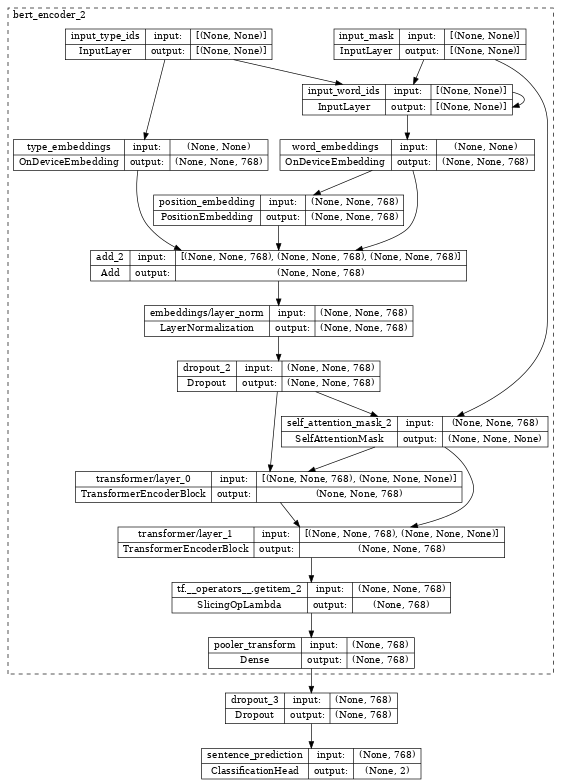Thanks for tuning in to Google I/O. View all sessions on demand

# Introduction to the TensorFlow Models NLP library

## Learning objectives

In this Colab notebook, you will learn how to build transformer-based models for common NLP tasks including pretraining, span labelling and classification using the building blocks from NLP modeling library.

## Install and import

### Install the TensorFlow Model Garden pip package

• `tf-models-official` is the stable Model Garden package. Note that it may not include the latest changes in the `tensorflow_models` github repo. To include latest changes, you may install `tf-models-nightly`, which is the nightly Model Garden package created daily automatically.
• `pip` will install all models and dependencies automatically.
````pip install tf-models-official`
```

### Import Tensorflow and other libraries

``````import numpy as np
import tensorflow as tf

from tensorflow_models import nlp
``````
```2022-12-17 12:11:31.934804: W tensorflow/compiler/xla/stream_executor/platform/default/dso_loader.cc:64] Could not load dynamic library 'libnvinfer.so.7'; dlerror: libnvinfer.so.7: cannot open shared object file: No such file or directory
2022-12-17 12:11:31.934918: W tensorflow/compiler/xla/stream_executor/platform/default/dso_loader.cc:64] Could not load dynamic library 'libnvinfer_plugin.so.7'; dlerror: libnvinfer_plugin.so.7: cannot open shared object file: No such file or directory
2022-12-17 12:11:31.934929: W tensorflow/compiler/tf2tensorrt/utils/py_utils.cc:38] TF-TRT Warning: Cannot dlopen some TensorRT libraries. If you would like to use Nvidia GPU with TensorRT, please make sure the missing libraries mentioned above are installed properly.
```

## BERT pretraining model

BERT (Pre-training of Deep Bidirectional Transformers for Language Understanding) introduced the method of pre-training language representations on a large text corpus and then using that model for downstream NLP tasks.

In this section, we will learn how to build a model to pretrain BERT on the masked language modeling task and next sentence prediction task. For simplicity, we only show the minimum example and use dummy data.

### Build a `BertPretrainer` model wrapping `BertEncoder`

The `nlp.networks.BertEncoder` class implements the Transformer-based encoder as described in BERT paper. It includes the embedding lookups and transformer layers (`nlp.layers.TransformerEncoderBlock`), but not the masked language model or classification task networks.

The `nlp.models.BertPretrainer` class allows a user to pass in a transformer stack, and instantiates the masked language model and classification networks that are used to create the training objectives.

``````# Build a small transformer network.
vocab_size = 100
network = nlp.networks.BertEncoder(
vocab_size=vocab_size,
# The number of TransformerEncoderBlock layers
num_layers=3)
``````

Inspecting the encoder, we see it contains few embedding layers, stacked `nlp.layers.TransformerEncoderBlock` layers and are connected to three input layers:

`input_word_ids`, `input_type_ids` and `input_mask`.

``````tf.keras.utils.plot_model(network, show_shapes=True, expand_nested=True, dpi=48)
````````````# Create a BERT pretrainer with the created network.
num_token_predictions = 8
bert_pretrainer = nlp.models.BertPretrainer(
network, num_classes=2, num_token_predictions=num_token_predictions, output='predictions')
``````
```WARNING:tensorflow:From /tmpfs/src/tf_docs_env/lib/python3.9/site-packages/official/nlp/modeling/models/bert_pretrainer.py:112: Classification.__init__ (from official.nlp.modeling.networks.classification) is deprecated and will be removed in a future version.
Instructions for updating:
WARNING:tensorflow:From /tmpfs/src/tf_docs_env/lib/python3.9/site-packages/official/nlp/modeling/models/bert_pretrainer.py:112: Classification.__init__ (from official.nlp.modeling.networks.classification) is deprecated and will be removed in a future version.
Instructions for updating:
```

Inspecting the `bert_pretrainer`, we see it wraps the `encoder` with additional `MaskedLM` and `nlp.layers.ClassificationHead` heads.

``````tf.keras.utils.plot_model(bert_pretrainer, show_shapes=True, expand_nested=True, dpi=48)
````````````# We can feed some dummy data to get masked language model and sentence output.
sequence_length = 16
batch_size = 2

word_id_data = np.random.randint(vocab_size, size=(batch_size, sequence_length))
type_id_data = np.random.randint(2, size=(batch_size, sequence_length))

outputs = bert_pretrainer(
sentence_output = outputs["classification"]
print(f'lm_output: shape={lm_output.shape}, dtype={lm_output.dtype!r}')
print(f'sentence_output: shape={sentence_output.shape}, dtype={sentence_output.dtype!r}')
``````
```lm_output: shape=(2, 8, 100), dtype=tf.float32
sentence_output: shape=(2, 2), dtype=tf.float32
```

### Compute loss

Next, we can use `lm_output` and `sentence_output` to compute `loss`.

``````masked_lm_ids_data = np.random.randint(vocab_size, size=(batch_size, num_token_predictions))
next_sentence_labels_data = np.random.randint(2, size=(batch_size))

mlm_loss = nlp.losses.weighted_sparse_categorical_crossentropy_loss(
predictions=lm_output,
sentence_loss = nlp.losses.weighted_sparse_categorical_crossentropy_loss(
labels=next_sentence_labels_data,
predictions=sentence_output)
loss = mlm_loss + sentence_loss

print(loss)
``````
```tf.Tensor(5.2983174, shape=(), dtype=float32)
```

With the loss, you can optimize the model. After training, we can save the weights of TransformerEncoder for the downstream fine-tuning tasks. Please see run_pretraining.py for the full example.

## Span labeling model

Span labeling is the task to assign labels to a span of the text, for example, label a span of text as the answer of a given question.

In this section, we will learn how to build a span labeling model. Again, we use dummy data for simplicity.

### Build a BertSpanLabeler wrapping BertEncoder

The `nlp.models.BertSpanLabeler` class implements a simple single-span start-end predictor (that is, a model that predicts two values: a start token index and an end token index), suitable for SQuAD-style tasks.

Note that `nlp.models.BertSpanLabeler` wraps a `nlp.networks.BertEncoder`, the weights of which can be restored from the above pretraining model.

``````network = nlp.networks.BertEncoder(
vocab_size=vocab_size, num_layers=2)

# Create a BERT trainer with the created network.
bert_span_labeler = nlp.models.BertSpanLabeler(network)
``````

Inspecting the `bert_span_labeler`, we see it wraps the encoder with additional `SpanLabeling` that outputs `start_position` and `end_position`.

``````tf.keras.utils.plot_model(bert_span_labeler, show_shapes=True, expand_nested=True, dpi=48)
````````````# Create a set of 2-dimensional data tensors to feed into the model.
word_id_data = np.random.randint(vocab_size, size=(batch_size, sequence_length))
type_id_data = np.random.randint(2, size=(batch_size, sequence_length))

# Feed the data to the model.
start_logits, end_logits = bert_span_labeler([word_id_data, mask_data, type_id_data])

print(f'start_logits: shape={start_logits.shape}, dtype={start_logits.dtype!r}')
print(f'end_logits: shape={end_logits.shape}, dtype={end_logits.dtype!r}')
``````
```start_logits: shape=(2, 16), dtype=tf.float32
end_logits: shape=(2, 16), dtype=tf.float32
```

### Compute loss

With `start_logits` and `end_logits`, we can compute loss:

``````start_positions = np.random.randint(sequence_length, size=(batch_size))
end_positions = np.random.randint(sequence_length, size=(batch_size))

start_loss = tf.keras.losses.sparse_categorical_crossentropy(
start_positions, start_logits, from_logits=True)
end_loss = tf.keras.losses.sparse_categorical_crossentropy(
end_positions, end_logits, from_logits=True)

total_loss = (tf.reduce_mean(start_loss) + tf.reduce_mean(end_loss)) / 2
print(total_loss)
``````
```tf.Tensor(3.37285, shape=(), dtype=float32)
```

With the `loss`, you can optimize the model. Please see run_squad.py for the full example.

## Classification model

In the last section, we show how to build a text classification model.

### Build a BertClassifier model wrapping BertEncoder

`nlp.models.BertClassifier` implements a [CLS] token classification model containing a single classification head.

``````network = nlp.networks.BertEncoder(
vocab_size=vocab_size, num_layers=2)

# Create a BERT trainer with the created network.
num_classes = 2
bert_classifier = nlp.models.BertClassifier(
network, num_classes=num_classes)
``````

Inspecting the `bert_classifier`, we see it wraps the `encoder` with additional `Classification` head.

``````tf.keras.utils.plot_model(bert_classifier, show_shapes=True, expand_nested=True, dpi=48)
````````````# Create a set of 2-dimensional data tensors to feed into the model.
word_id_data = np.random.randint(vocab_size, size=(batch_size, sequence_length))
type_id_data = np.random.randint(2, size=(batch_size, sequence_length))

# Feed the data to the model.
print(f'logits: shape={logits.shape}, dtype={logits.dtype!r}')
``````
```logits: shape=(2, 2), dtype=tf.float32
```

### Compute loss

With `logits`, we can compute `loss`:

``````labels = np.random.randint(num_classes, size=(batch_size))

loss = tf.keras.losses.sparse_categorical_crossentropy(
labels, logits, from_logits=True)
print(loss)
``````
```tf.Tensor([0.3355983 1.2492478], shape=(2,), dtype=float32)
```

With the `loss`, you can optimize the model. Please see the Fine tune_bert notebook or the model training documentation for the full example.

[]
[]